# tkinter之按钮Button组件讲解

### 创建一个默认的按钮

```from tkinter import *
root=Tk()
but1=Button(root,text="确定") # 定义按钮
root.mainloop()```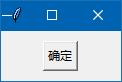### 属性：text,wraplength,justify

1．text ：按钮上显示的文本。

2．wraplength 决定第一行文本的长度，单位是像素。

3．justify 决定多行显示文本对齐方式。

```a='确定选\n择合适\n选项'
but1=Button(root,text=a) # 定义按钮

```a='''确定选

but1=Button(root,text=a) # 定义按钮

```a='确定选择合适选项'
but1=Button(root,text=a,wraplength=40) # 定义按钮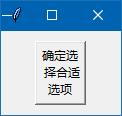```a='确定选择合适选项'
but1=Button(root,text=a,wraplength=40,justify='left') # 定义按钮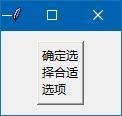### 属性：relief,font

relief : 按钮的样式。按钮的样式跟标签完全一样。所有样式如下：

#  flat  groove  raised  ridge   solid   sunken

#  平    边凹       凸       边凸      黑框       凹

上一篇文章，我们把标签伪装成按钮的样子，同样，我们也可以把按钮伪装成标签（　relief='flat’），只是这个伪装成标签的按钮是支持鼠标点击的。

```a='确定选择合适选项'
but1=Button(root,text=a,wraplength=40,justify='left',relief='flat') # 定义按钮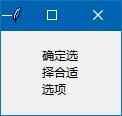font : 字体设置。跟标签一样，可以用元组方法来设置字体，我们来回顾一下：

```from tkinter import *
root=Tk()
but1=Button(root,text='确定',font=('黑体',12,'bold','italic','underline','overstrike')) # 定义按钮
root.mainloop()```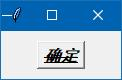### 属性：fg,bg

fg,  foreground ：字体颜色

bg,  background ：按钮背景颜色

```from tkinter import *
root=Tk()
but1=Button(root,text='确定',fg='white',bg='green') # 定义按钮
root.mainloop()```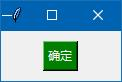```from tkinter import *
root=Tk()
root.mainloop()```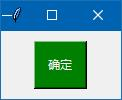### 属性：widht,height

width, height 以字符为单位为决定按钮的宽和高，如果按钮内容有图像，那单位应该是像素。

注意：字符单位是英文字符单位，中文字符要乘2，可能还不太准确。

```from tkinter import *
root=Tk()
but1=Button(root,text='确定',fg='white',bg='green',width=8,height=2) # 定义按钮
root.mainloop()```

width=8,height=2 说明这个按钮的宽，可以容纳8个英文字符，高可以容纳2行字符。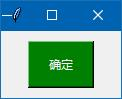activebackground ： 当按钮组件处于活动状态时的背景颜色。

activeforeground  ： 当按钮组件处于活动状态时的字体颜色。

state : 　组件的状态

有时，我们希望鼠标点击按钮时，有颜色方面的变化，就可以用到上述2个参数。让按钮处于活动状态时，背景色，字体颜色都会有变化，按钮状态可以用鼠标直接点击改变，也可以用 state() 方法来设置。

```from tkinter import *
root=Tk()
but1=Button(root,text='确定',activebackground='red',activeforeground='white') # 定义按钮
but1['state']='active'  # 设置活动状态
root.mainloop()```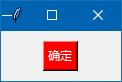but1['state']='active'

but1['state']='disabled'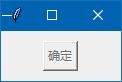### 设置按钮的焦点

```from tkinter import *
root=Tk()
but1.focus_set()  # 设置焦点
root.mainloop()```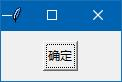### 属性：image

按钮也可以像标签一样显示图像，而且相关语法跟标签完全一样，在这里，我们再把相关知识回顾一下。

先在代码目录里准备一个图片文件：horn.png　（一个喇叭图）　　　　丶丌皛

```from tkinter import *
root=Tk()
img1=PhotoImage(file='horn.png') # 先把图像转为图像对象
but1=Button(root,text='确定',image=img1) # image=图像对象
root.mainloop()```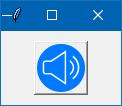```from tkinter import *
root=Tk()
img1=PhotoImage(file='horn.png') # 先把图像转为图像对象
but1=Button(root,text='确定',image=img1,compound='left') # image=图像对象
root.mainloop()```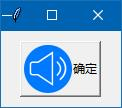### 自定义外观的按钮

有朋友想自己做一个按钮，自己先准备一个png图片文件，这个png图像四周圆角部分是透明的。代码如下：

```from tkinter import *
root=Tk()
# root['bg']='yellow' # 这里可以设置窗体背景颜色
img1=PhotoImage(file='button.png')
but1=Button(root,text='退出',image=img1,compound='center')
but1.config(relief='flat',bd=0,bg=root['bg'],activebackground=root['bg'])  # 这个按钮必须要设置这些属性才行
root.mainloop()```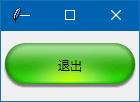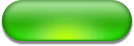tkinter按钮改变外观的说明：

这个按钮必须要设置：样式为flat，边宽为0，按钮背景颜色以及活动状态下的背景颜色必须跟窗体的背景颜色统一才可以做一个自定义的按钮。

在标签里有一个属性： textvariable 没有讲解，按钮也有这个参数，这里也不讲解，以后会在讲解变量里，专门讲解。

### 属性：command

下面要讲解按钮最重要的一个参数：

command : 指定按钮点击以后要执行的动作(回调函数)。

command参数有几种写法：

第1种方法：

```from tkinter import *
root=Tk()
but1=Button(root,text='退出',command=root.destroy)  # 点击退出
root.mainloop()```

第2种方法：

```from tkinter import *
def callback():
print('你好')
root=Tk()
but1=Button(root,text='退出',command=callback)  # 点击退出
root.mainloop()```

第3种方法：

```from tkinter import *
def callback(a):
print('你好',a)
root=Tk()
but1=Button(root,text='退出',command=lambda : callback('张三'))  # 点击退出
root.mainloop()```

```def callback(a,b):
print('你好',a,b)```

Buttom 按钮组件就讲解到这，下一篇文章写 Entry 单行文本框组件。

<< 上一篇 下一篇 >>